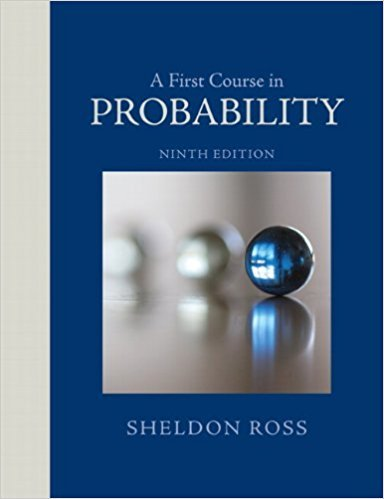×
Get Full Access to A First Course In Probability - 9 Edition - Chapter 2 - Problem 33p
Get Full Access to A First Course In Probability - 9 Edition - Chapter 2 - Problem 33p

×

# A forest contains 20 elk, of which 5 are captured, tagged,ISBN: 9780321794772 63

## Solution for problem 33P Chapter 2

A First Course in Probability | 9th Edition

• Textbook Solutions
• 2901 Step-by-step solutions solved by professors and subject experts
• Get 24/7 help from StudySoup virtual teaching assistantsA First Course in Probability | 9th Edition

4 5 1 335 Reviews
26
2
Problem 33P

Problem 33P

A forest contains 20 elk, of which 5 are captured, tagged, and then released. A certain time later, 4 of the 20 elk are captured. What is the probability that 2 of these 4 have been tagged? What assumptions are you making?

Step-by-Step Solution:

Solution:

Step 1 of 2:

It is given that a forest contains 20 elk, out of which 5 are captured, tagged and are released.

Also, it is given that after some times, 4 of 20 elk are captured.

Using this we need to find the probability that 2 out of the 4 have been tagged.Also we have to mention the assumptions made.

Step 2 of 2

##### ISBN: 9780321794772

A First Course in Probability was written by and is associated to the ISBN: 9780321794772. This textbook survival guide was created for the textbook: A First Course in Probability , edition: 9. The answer to “A forest contains 20 elk, of which 5 are captured, tagged, and then released. A certain time later, 4 of the 20 elk are captured. What is the probability that 2 of these 4 have been tagged? What assumptions are you making?” is broken down into a number of easy to follow steps, and 42 words. Since the solution to 33P from 2 chapter was answered, more than 328 students have viewed the full step-by-step answer. This full solution covers the following key subjects: captured, tagged, elk, later, assumptions. This expansive textbook survival guide covers 4 chapters, and 469 solutions. The full step-by-step solution to problem: 33P from chapter: 2 was answered by , our top Statistics solution expert on 08/11/17, 08:57AM.

Unlock Textbook Solution